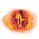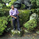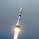6390 vues
Hello everyone,

Quick script to check the PEG Ratio.

What is PEG Ratio?
The price/ earnings to growth ratio (PEG Ratio) is a stock's price-to-earnings (P/E) ratio divided by the growth rate of its earnings for a specified time period. The PEG ratio is used to determine a stock's value while also factoring in the company's expected earnings growth, and is thought to provide a more complete picture than the more standard P/E ratio .

A PEG Ratio greater than 1.0 means that the stock is overvalued, while below 1.0 means is is undervalued. When the PEG Ratio is exactly 1.0, then the stock is trading at fair valuation.

Formula:
PEG Ratio = (Price / EPS ) / EPS Growth

Examples:

Company A:
• Price per share = \$46
• EPS this year = \$2.09
• EPS last year = \$1.74
Company B
• Price per share = \$80
• EPS this year = \$2.67
• EPS last year = \$1.78

Company A
• P/E ratio = \$46 / \$2.09 = 22
• Earnings growth rate = (\$2.09 / \$1.74) - 1 = 20%
• PEG ratio = 22 / 20 = 1.1
Company B
• P/E ratio = \$80 / \$2.67 = 30
• Earnings growth rate = (\$2.67 / \$1.78) - 1 = 50%
• PEG ratio = 30 / 50 = 0.6

The company A is overvalued whiled the B is undervalued.

In this script an overvalued stock is considered when the PEG Ratio is above 1.1, while it is 0.9 for an undervalued stock.

Only works with Stocks.

Script open-source

Dans le plus pur esprit de TradingView, l'auteur de ce texte l'a publié en open-source, afin que les traders puissent le comprendre et le vérifier. Bravo à l'auteur ! Vous pouvez l'utiliser gratuitement, mais la réutilisation de ce code dans une publication est régie par les règles internes. Vous pouvez le mettre en favori pour l'utiliser sur un graphique.

Vous voulez utiliser ce script sur un graphique ?

## CommentairesDo you know of a way to calculate this "manually"? Price is a bar-by-bar change so presumably this can be calculated on a bar-by-bar basis (not just through FQ/FY financials).
RépondreTomotto
@Tomotto, mine is set to 4, but I'm still getting the resolve error. Do you know how I can fix this?
RépondreIntermarketics
@Intermarketics, Can you tell me which stock you're trying to apply this script on?
RépondreIntermarketics
@Intermarketics, couldn't find anything yet. I'll continue to look
RépondreIntermarketics
@Intermarketics, Never mind, I solved the issue. Thanks though
Répondre